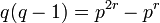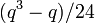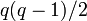# Subgroup structure of special linear group:SL(2,5)

View subgroup structure of particular groups | View other specific information about special linear group:SL(2,5)

This article describes the subgroup structure of special linear group:SL(2,5), which is the special linear group of degree two over field:F5. The group has order 120.

## Family contexts

Family name Parameter values General discussion of subgroup structure of family
special linear group of degree two field:F5, i.e., the group$SL(2,5)$ subgroup structure of special linear group of degree two over a finite field
double cover of alternating group$2 \cdot A_n$ degree$n = 5$, i.e., the group$2 \cdot A_5$ subgroup structure of double cover of alternating group

## Tables for quick information

FACTS TO CHECK AGAINST FOR SUBGROUP STRUCTURE: (finite group)
Lagrange's theorem (order of subgroup times index of subgroup equals order of whole group, so both divide it), |order of quotient group divides order of group (and equals index of corresponding normal subgroup)
Sylow subgroups exist, Sylow implies order-dominating, congruence condition on Sylow numbers|congruence condition on number of subgroups of given prime power order
normal Hall implies permutably complemented, Hall retract implies order-conjugate

### Quick summary

Item Value
number of subgroups 76
number of conjugacy classes of subgroups 12
number of automorphism classes of subgroups 12
isomorphism classes of Sylow subgroups, corresponding fusion systems, and Sylow numbers 2-Sylow: quaternion group (order 8) with its non-inner fusion system (see non-inner fusion system for quaternion group), Sylow number 5
3-Sylow: cyclic group:Z3 with its non-inner fusion system, Sylow number 10
5-Sylow: cyclic group:Z5, Sylow number 6
Hall subgroups Other than the whole group, trivial subgroup, and Sylow subgroups, there is a$\{ 2,3 \}$-Hall subgroup of order 24 (SL(2,3) in SL(2,5)). There is no$\{ 2,5 \}$-Hall subgroup or$\{ 3,5 \}$-Hall subgroup.
maximal subgroups There are maximal subgroups of order 12 (index 10), order 20 (index 6) and order 24 (index 5).
normal subgroups The only proper nontrivial normal subgroup is center of special linear group:SL(2,5), which is isomorphic to cyclic group:Z2 and the quotient group is isomorphic to alternating group:A5.

## Defining functions

### Subgroup-defining functions and associated quotient-defining functions

Subgroup-defining function What it means Value as subgroup Value as group Order Associated quotient-defining function Value as group Order (= index of subgroup)
center elements that commute with every group element center of special linear group:SL(2,5) cyclic group:Z2 2 inner automorphism group alternating group:A5 60
derived subgroup subgroup generated by all commutators whole group special linear group:SL(2,5) 120 abelianization trivial group 1
perfect core the subgroup at which the derived series stabilizes whole group special linear group:SL(2,5) 120  ? trivial group 1
hypocenter the subgroup at which the lower central series stabilizes whole group special linear group:SL(2,5) 120  ? trivial group 1
hypercenter the subgroup at which the upper central series stabilizes center of special linear group:SL(2,5) cyclic group:Z2 2  ? alternating group:A5 60
Frattini subgroup intersection of all maximal subgroups center of special linear group:SL(2,5) cyclic group:Z2 2 Frattini quotient alternating group:A5 60
Jacobson radical intersection of all maximal normal subgroups center of special linear group:SL(2,5) cyclic group:Z2 2  ? alternating group:A5 60
socle join of all minimal normal subgroups center of special linear group:SL(2,5) cyclic group:Z2 2 socle quotient alternating group:A5 60
Baer norm intersection of normalizers of all subgroups center of special linear group:SL(2,5) cyclic group:Z2 2  ? alternating group:A5 60
join of all abelian normal subgroups subgroup generated by all the abelian normal subgroups center of special linear group:SL(2,5) cyclic group:Z2 2  ? alternating group:A5 60
Fitting subgroup subgroup generated by all the nilpotent normal subgroups center of special linear group:SL(2,5) cyclic group:Z2 2  ? alternating group:A5 60
solvable radical subgroup generated by all the solvable normal subgroups center of special linear group:SL(2,5) cyclic group:Z2 2  ? alternating group:A5 60
socle over solvable radical its quotient by the solvable radical of the group is the socle of the quotient of the group by the solvable radical whole group special linear group:SL(2,5) 120  ? trivial group 1
permutation kernel too complicated to describe here whole group special linear group:SL(2,5) 120  ? trivial group 1

## Sylow subgroups

Compare and contrast with subgroup structure of special linear group of degree two over a finite field#Sylow subgroups

We are considering the group$SL(2,q)$ with$q = p^r$ a prime power,$q = 5, p = 5, r = 1$. The prime$p = 5$ is the characteristic prime.

### Sylow subgroups for the prime 5

The prime 5 is the characteristic prime$p$, so we compare with the general information on$p$-Sylow subgroups of$SL(2,q)$.

Item Value for$SL(2,q)$, generic$q$ Value for$SL(2,5)$ (so$q = p = 5, r = 1$)
order of$p$-Sylow subgroup$q$ 5
index of$p$-Sylow subgroup$q^2 - 1$ 24
explicit description of one of the$p$-Sylow subgroups unitriangular matrix group of degree two:$\{ \begin{pmatrix} 1 & b \\ 0 & 1 \\\end{pmatrix} \mid b \in \mathbb{F}_q \}$ See 5-Sylow subgroup of special linear group:SL(2,5)
isomorphism class of$p$-Sylow subgroup additive group of the field$\mathbb{F}_q$, which is an elementary abelian group of order$p^r$ cyclic group:Z5
explicit description of$p$-Sylow normalizer Borel subgroup of degree two:$\{ \begin{pmatrix} a & b \\ 0 & a^{-1} \\\end{pmatrix} \mid a \in \mathbb{F}_q^\ast, b \in \mathbb{F}_q \}$ See 5-Sylow normalizer of special linear group:SL(2,5)
isomorphism class of$p$-Sylow normalizer It is the external semidirect product of$\mathbb{F}_q$ by the multiplicative group of$\mathbb{F}_q^\ast$ where the latter acts on the former via the multiplication action of the square of the acting element.
For$p = 2$ (so$q = 2,4,8,\dots$), it is isomorphic to the general affine group of degree one$GA(1,q)$.
For$q = 3$, it is cyclic group:Z6 and for$q = 5$, it is dicyclic group:Dic20.
dicyclic group:Dic20
order of$p$-Sylow normalizer$q(q - 1) = p^{2r} - p^r$ 20$p$-Sylow number (i.e., number of$p$-Sylow subgroups) = index of$p$-Sylow normalizer$q + 1$ (congruent to 1 mod p, as expected from the congruence condition on Sylow numbers) 6

### Sylow subgroups for the prime 2

We are in the subcase where$\ell = 2$ ($\ell$ being the prime for which we are taking Sylow subgroups) and$q \equiv 5 \pmod 8$.

Item Value for$\ell = 2, q \equiv 5 \pmod 8$ Value for$\ell = 2, q = p = 5, r = 1$ (our case)
order of 2-Sylow subgroup 8 8
index of 2-Sylow subgroup$(q^3 - q)/8$ 15
explicit description of one of the 2-Sylow subgroups Since multiplicative group of a finite field is cyclic,$\mathbb{F}_q^\ast$ is cyclic of order$q - 1$. Let$H$ be its unique subgroup of order 4. Then, the 2-Sylow subgroup is$\{ \begin{pmatrix} a & 0 \\ 0 & a^{-1} \\\end{pmatrix} \mid a \in H \} \cup \{ \begin{pmatrix} 0 & a \\ -a^{-1} & 0 \\\end{pmatrix} \mid a \in H \}$ 2-Sylow subgroup of special linear group:SL(2,5)
isomorphism class of 2-Sylow subgroup quaternion group quaternion group
explicit description of 2-Sylow normalizer PLACEHOLDER FOR INFORMATION TO BE FILLED IN: [SHOW MORE] SL(2,3) in SL(2,5)
isomorphism class of 2-Sylow normalizer special linear group:SL(2,3) special linear group:SL(2,3)
order of 2-Sylow normalizer 24 24
2-Sylow number (i.e., number of 2-Sylow subgroups) = index of 2-Sylow normalizer$(q^3 - q)/24$ 5

### Sylow subgroups for the prime 3

Here,$\ell = 3$ and we are interested in the$\ell$-Sylow subgroups.

We are in the subcase$\ell$ is an odd prime dividing$q + 1$. Suppose$\ell^t$ is the largest power of$\ell$ dividing$q + 1$. In our case,$t = 1$.

Item Value for generic$\ell, t, p, q, r$ Value for$\ell = 3, t = 1, p = q = 5, r = 1$
order of$\ell$-Sylow subgroup$\ell^t$ 3
index of$\ell$-Sylow subgroup$(q^3 - q)/\ell^t$ 40
explicit description of one of the$\ell$-Sylow subgroups Since multiplicative group of a finite field is cyclic,$\mathbb{F}_{q^2}^\ast$ is cyclic of order$q^2 - 1$. Further, via the action on a two-dimensional vector space over$\mathbb{F}_q$, we can embed$\mathbb{F}_{q^2}^\ast$ inside$GL(2,q)$. The image of the$\ell$-Sylow subgroup of$\mathbb{F}_{q^2}^\ast$ in$GL(2,q)$ actually lands inside$SL(2,q)$, and this image is a$\ell$-Sylow subgroup of$SL(2,q)$ 3-Sylow subgroup of special linear group:SL(2,5)
isomorphism class of$\ell$-Sylow subgroup cyclic group of order$\ell^t$ cyclic group:Z3
explicit description of$\ell$-Sylow normalizer PLACEHOLDER FOR INFORMATION TO BE FILLED IN: [SHOW MORE]
isomorphism class of$\ell$-Sylow normalizer Case$p = 2$: dihedral group of order$2(q + 1)$
Case$p \ne 2$: dicyclic group of order$2(q + 1)$
dicyclic group:Dic12
order of$\ell$-Sylow normalizer$2(q + 1)$ 12$\ell$-Sylow number (i.e., number of$\ell$-Sylow subgroups) = index of$\ell$-Sylow normalizer$q(q - 1)/2$ (congruent to 1 mod$\ell$, as expected from the congruence condition on Sylow numbers) 10